Learning Library

Subtraction on the Farm

Math's not so baa-aaa-ad! These farm-themed worksheets keep subtraction interesting.

Click on a worksheet in the set below to see more info or download the PDF.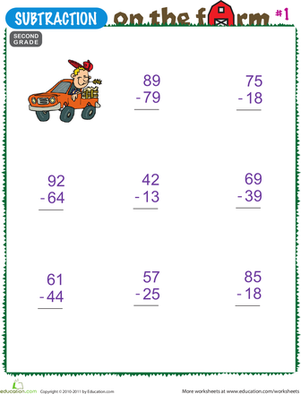Subtraction on the Farm #1

Help your 2nd grader practice two-digit subtraction with this farm-themed worksheet. Kids will solve subtraction problems alongside a friendly farmer.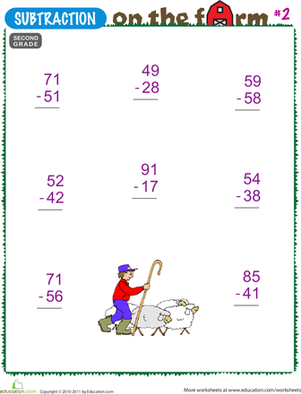Subtraction on the Farm #2

2nd grade mathematicians will practice two-digit subtraction with this farm-themed worksheet. Subtract with borrowing alongside a farmer herding sheep.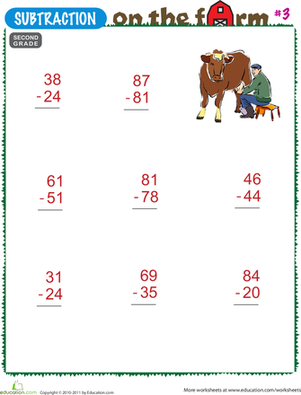Subtraction on the Farm #3

This 2nd grade math worksheet helps kids practice two-digit subtraction. This farm-themed worksheet features a set of math exercises and a farmer milking a cow.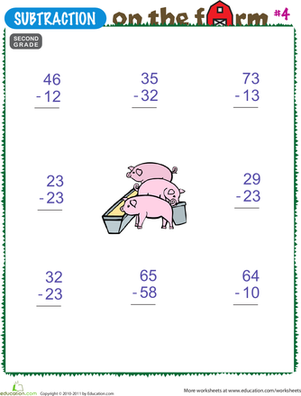Subtraction on the Farm #4

Practice two-digit subtraction with this farm-themed worksheet. 2nd graders will solve math problems alongside a friendly group of pigs.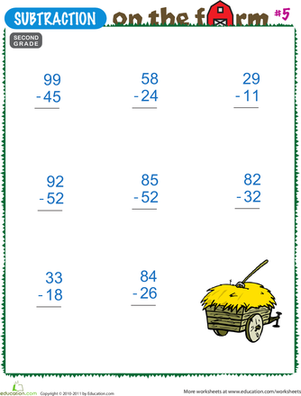Subtraction on the Farm #5

2nd grade students will enjoy this farm-themed math worksheet. Solve two-digit subtraction problems alongside a bale of hay.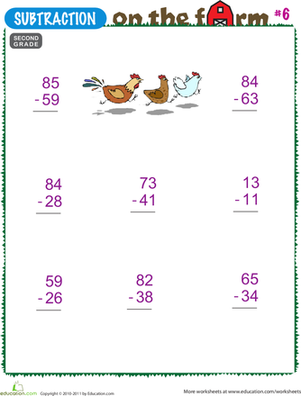Subtraction on the Farm #6

Cluck your way through two-digit subtraction with this farm-themed worksheet. Chickens help your 2nd grader solve math problems.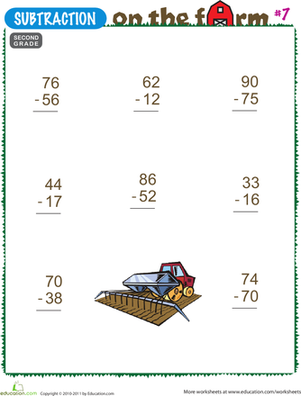Subtraction on the Farm #7

2nd grade students will solve two-digit subtraction problems with this farm-themed worksheet. This worksheet includes a set of math problems and a farm picture.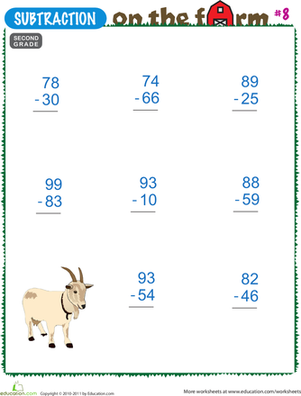Subtraction on the Farm #8

Have fun with math using this farm-themed worksheet. 2nd graders will solve two-digit subtraction problems alongside a farmer's goat.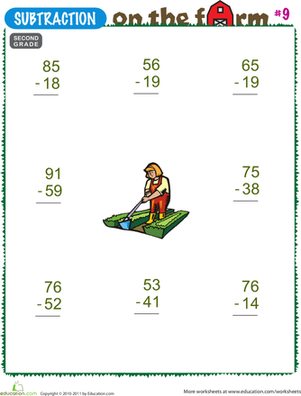Subtraction on the Farm #9

This 2nd grade worksheet gets kids to solve two-digit subtraction problems. Solve math problems in this farm-themed worksheet alongside a farmer.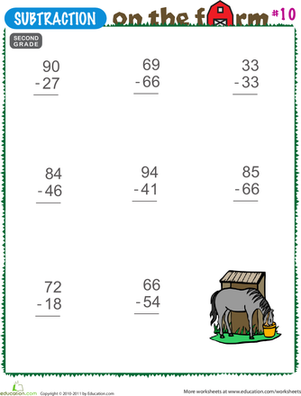Subtraction on the Farm #10

This farm-themed math worksheet makes two-digit subtraction fun. 2nd graders will solve problems next to a farmer's horse.

Want to download this whole set as a single PDF?

Create new collection

0

New Collection>

0 items

What could we do to improve Education.com?

Please note: Use the Contact Us link at the bottom of our website for account-specific questions or issues.

What would make you love Education.com?